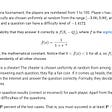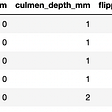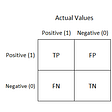# In Depth: Parameter tuning for SVC

In this post we will explore the most important parameters of Sklearn SVC classifier and how they impact our model in term of overfitting.

Support Vectors Classifier tries to find the best hyperplane to separate the different classes by maximizing the distance between sample points and the hyperplane.

We will use the Iris data from sklearn.

`import numpy as npimport matplotlib.pyplot as pltfrom sklearn import svm, datasets%matplotlib inline# import some data to play withiris = datasets.load_iris()X = iris.data[:, :2] # we only take the first two features. We could # avoid this ugly slicing by using a two-dim datasety = iris.target`

We use the same plotting script for our plots.

`def plotSVC(title):  # create a mesh to plot in  x_min, x_max = X[:, 0].min() — 1, X[:, 0].max() + 1  y_min, y_max = X[:, 1].min() — 1, X[:, 1].max() + 1  h = (x_max / x_min)/100  xx, yy = np.meshgrid(np.arange(x_min, x_max, h), np.arange(y_min, y_max, h)) plt.subplot(1, 1, 1) Z = svc.predict(np.c_[xx.ravel(), yy.ravel()]) Z = Z.reshape(xx.shape) plt.contourf(xx, yy, Z, cmap=plt.cm.Paired, alpha=0.8) plt.scatter(X[:, 0], X[:, 1], c=y, cmap=plt.cm.Paired) plt.xlabel(‘Sepal length’) plt.ylabel(‘Sepal width’) plt.xlim(xx.min(), xx.max()) plt.title(title) plt.show()`

# Kernel

kernel parameters selects the type of hyperplane used to separate the data. Using ‘linear’ will use a linear hyperplane (a line in the case of 2D data). ‘rbf’ and ‘poly’ uses a non linear hyper-plane

`kernels = [‘linear’, ‘rbf’, ‘poly’]for kernel in kernels:  svc = svm.SVC(kernel=kernel).fit(X, y)  plotSVC(‘kernel=’ + str(kernel))`

# gamma

gamma is a parameter for non linear hyperplanes. The higher the gamma value it tries to exactly fit the training data set

`gammas = [0.1, 1, 10, 100]for gamma in gammas:   svc = svm.SVC(kernel=’rbf’, gamma=gamma).fit(X, y)   plotSVC(‘gamma=’ + str(gamma))`

We can see that increasing gamma leads to overfitting as the classifier tries to perfectly fit the training data

# C

C is the penalty parameter of the error term. It controls the trade off between smooth decision boundary and classifying the training points correctly.

`cs = [0.1, 1, 10, 100, 1000]for c in cs:   svc = svm.SVC(kernel=’rbf’, C=c).fit(X, y)   plotSVC(‘C=’ + str(c))`

Increasing C values may lead to overfitting the training data.

# degree

degree is a parameter used when kernel is set to ‘poly’. It’s basically the degree of the polynomial used to find the hyperplane to split the data.

`degrees = [0, 1, 2, 3, 4, 5, 6]for degree in degrees:   svc = svm.SVC(kernel=’poly’, degree=degree).fit(X, y)   plotSVC(‘degree=’ + str(degree))`

Using degree=1 is the same as using a ‘linear’ kernel. Also, increasing this parameters leads to higher training times.

The inDepth series investigates how model parameters affect performance in term of overfitting and underfitting. You can check parameter tuning for tree based models like Decision Tree , Random Forest, Gradient Boosting and KNN.

--

--

--

## More from All things AI

This publication is dedicated to all things AI. Posts will cover Data Science, Machine Learning, Big Data and AI related

## Treemaps & Sunburst Charts with Plotly## Gauge & Bullet Charts## Collect Data with Scale in Mind## DDDM in times of uncertainty, 5 rules.## Google Code Jam 2021- Cheating Detection (Qualification Round Question #5)## The EDA Theoretical Guide## Practical Data Analysis With Pandas and Seaborn/MatplotlibAspiring Great Data Scientist https://maviator.github.io

## Electrical Power Systems — exploring smart cities via Data Science## Algorithms used by Boosting## ML Hands-On Intro: Build a Classifier## Evaluating Classification Models, Confusion Matrix